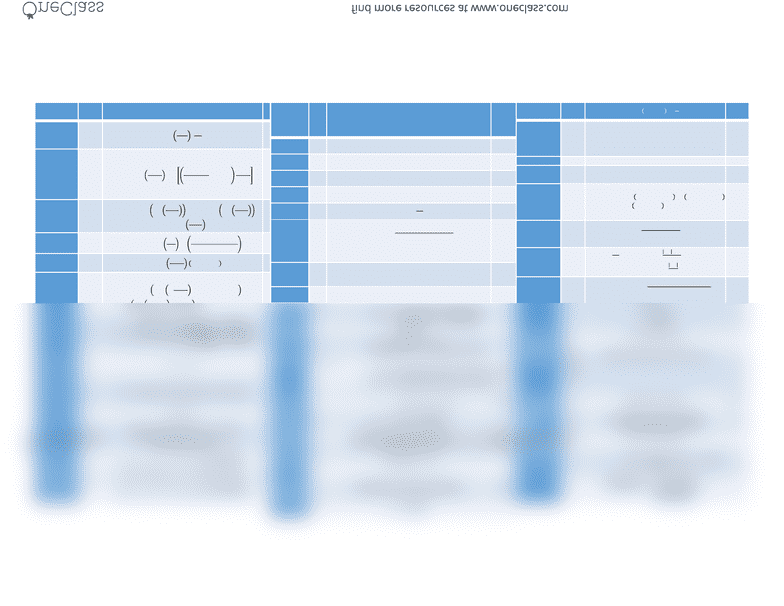Study Guides (380,000)
CA (150,000)
Carleton (5,000)
ENVE (10)
Final

ENVE 4105 Final: GBD Exam Sheet

Department
Environmental Engineering
Course Code
ENVE 4105
Professor
Liam O' Brien
Study Guide
Final

This preview shows half of the first page. to view the full 2 pages of the document.Variable Symbol Formulas
Surface Tilt
Slope Angle β30º
° =
°
Declination ɗ = 23.45°
180°   + 284
365 × 360°
180°
Equation of
Time ET  = 9.87sin 4 
 − 7.53cos 2 
 -
1.5sin(2 
 )
Assumed Solar
Time AST  =  + 
60 +4 ( − )
60
The Hour Angle hℎ = 15°
180°  − 12
Solar Altitude α
= sin  
180° ×   ×  ℎ
+ sin 
180° × sin
Solar Azimuth Ф = cos  × 
180° − 
  ×
180°
Surface Solar
Azimuth γ =  − Ψ
Solar Incident
Angle θ = coscos  cos sin + sin  cos 
Zenith zΖ =
2− 
I direct on
Surface
   =   × 
IF 90<G3<-90, then   = 0
(in this equation only)
I diffuse on
Surface    =  1 + cos 
2
I ground =   ×   + 
I ground
Reflected   =   + 1 + cos
2
Irradiance   =    +    +  
Annual net
heat gain
E ∙ ∑[()  ( )]
Net Heat gain q ∙ [()  ( )]
SHGCw(AfSHGCf+AeogSHGCeog+AcogSHGCcog)/(Af+Aeog+Acog)
Uw(AfUf+ AeogUeog + AcogUcog)/(Af+Aeog+Acog)
Resistance Rsi  =

(W/m2K)
Mass Balance
Equation  = − /
Assume P=1, k=0
= #   ×  
UA (specific heat)(density of air)(Q)(V/3600s/hr) W/mK
Percent
Outdoor Air
%OA % = , − ,
, − ,
Infiltration mCpΔT
Ventilation
Rate
Vbz  = + 
    
Relative
humidity ϕϕ
=     
    
Vapour
Pressure  =   + Pascals
Water
Content
W = .622
= . 

Enthalpy hh= ℎ + ℎ  kJ/kgda
Sensible Heat
Loss
qq = mCp(Tin - Tout)Watts
Surface Heat
Exchange
qq = hcA(Tsurf - Tair)
HDD Annual
Heat Loss (HDD)(U)(A2)(1/24hrs)(1/1000W) KWh
Heat Flux q = − 
Thermal Loss
Calculations  = − ×
Life Cycle
Energy
LCE LCE = embodied energy + life (years) x energy
use/year + demolition energy OR
recycling/reuse energy
Kelvin K C + 273.15
RSI 5.67 R (hr∙ft2°F/Btu) M2K/W
Human
Energy
Balance
−  =  +  + 
= +  +  +  + 
+  + 
Operative
Temperature = + ℎ
+ ℎ
      
Coeffecient of
Performance
COP  =
 =
 =
Illuminance E = Φ
  
 =
= 
Light Loss
factor
LLF LLF = BOF x LLD x BF
Burnout
Factor
Burnou
t
Factor
=100-(% of bulbs likely to be burnt out)
Light Power
Intensity
LPI Light power intensity W/m2
Daylight
Factor
DF DF=      
   
%
Contaminant
Concentration
ppm Ppm x 24.45= mg/m3
Electricity
cost = ℎ × \$
ℎ +  × \$/
 
  =  
  ×
 
 